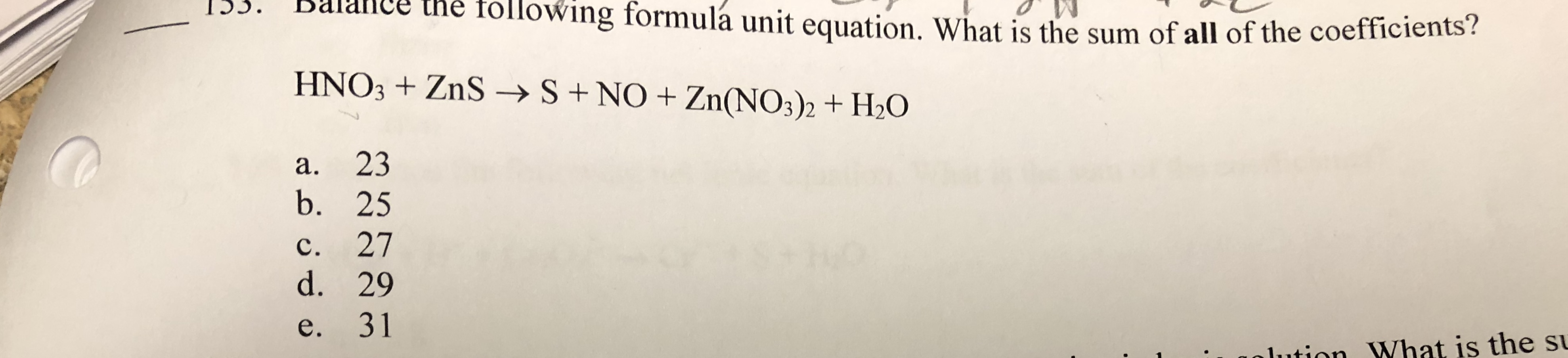# I.S. Bhe tollowing formulá unit equation. What is the sum of all of the coefficients?HNO3 ZnS- S+ NO Zn(NOs)2 + 20a. 23b. 25c. 27d. 29e. 31ution What is the s

Question
1 viewshelp_outlineImage TranscriptioncloseI.S. B he tollowing formulá unit equation. What is the sum of all of the coefficients? HNO3 ZnS- S+ NO Zn(NOs)2 + 20 a. 23 b. 25 c. 27 d. 29 e. 31 ution What is the s fullscreen
check_circle

Step 1

A balanced equation obeys the law of conservation of mass where the number of atoms remains constant throughout the reaction. In a balanced equation the number of atoms of each element on the reactant side and product side should be equal.

Step 2

In the given reaction, the number of atoms of each element on the right side and left side are not equal. The number of atoms is made constant by giving appropriate coefficient as given.

Step 3

Coefficient is a number placed before a formula in a balanced chemical equation that refers to the numbe...

### Want to see the full answer?

See Solution

#### Want to see this answer and more?

Solutions are written by subject experts who are available 24/7. Questions are typically answered within 1 hour.*

See Solution
*Response times may vary by subject and question.
Tagged in

### General Chemistry